# Practice Exam 2 Hints - You should attempt to work the...

• Test Prep
• 4
• 100% (1) 1 out of 1 people found this document helpful

This preview shows page 1 - 2 out of 4 pages.

The preview shows page 1 - 2 out of 4 pages.
You should attempt to work the problems on the practice exam on your own first. Consult the followinghints only if you are stuck and cannot figure out how to proceed.Practice Exam 2 Hints1.Write out the total ionic equation for the reaction, and see which ion(s) appear on both sides.2.How would you determine the products of a double-displacement reaction? What precipitatewill form (based on the solubility rule)? (Also, refer to hint for problem #1)3.What do your solubility rules regarding group I metal ions, nitrates, and sulfides tell you?4.Remember that the charge of a pure element is 0. OIL RIG. LEO GER.5.H always has a charge of +1 except in metal hydride. Group 7 nonmetals usually have a chargeof -1. The substance that is oxidized is the reducing agent. (Also, refer to hint for problem #4)6.The elements on the left of the activity series are stronger (more reactive) than those on theright.7.The sum of oxidation states of all elements in this ion must equal -2.8.Look up the definitions of base and acid.9.Decomposition is the general splitting of a compound. The answer to this question is morespecific.10.In order to calculate mass, we need to know what quantity first? How can we calculate it usingmolarity and volume?11.how many mL does the final solution have?12.– During dilution, the number of moles of solute does not change, i.e., the number of moles of
solute before dilution = the number of moles of solute after dilution– if molarity changes by 1/10, and moles remains the same, what has to happen to the volumeof solution?13.Solubility rules: what types of compounds are typically soluble? Do any of these compoundsviolate a solubility rule?14.Is this a double or single replacement reaction? (Solubility rules apply to double displacementreactions; activity series apply to single-replacement reactions.)15.Write the balanced chemical equation for the reaction first. What is the molar ration betweensulfuric acid and sodium hydroxide in the reaction? Can you calculate the moles of NaOH? If so,

Course Hero member to access this document

##### We have textbook solutions for you!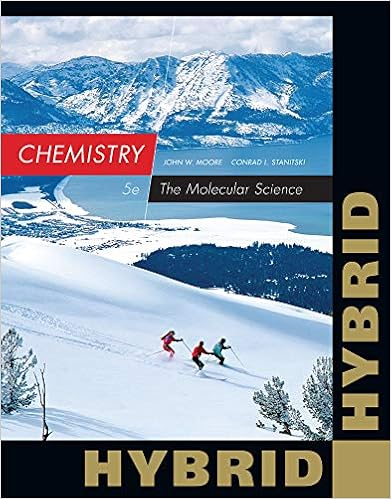The document you are viewing contains questions related to this textbook.
Chapter 3 / Exercise 3.27
Chemistry: The Molecular Science
Moore/StanitskiExpert Verified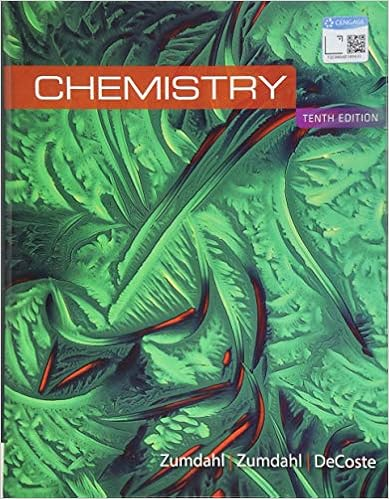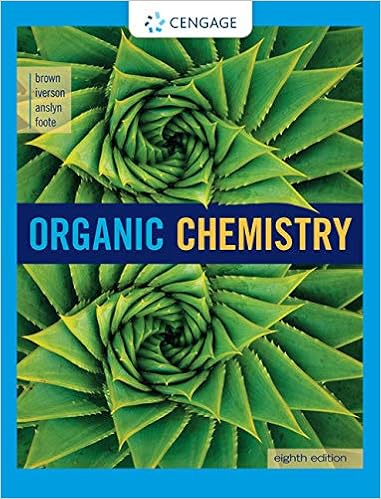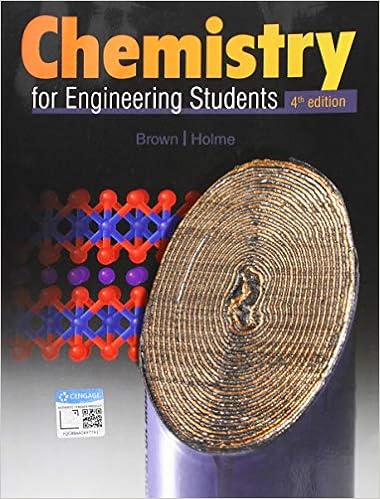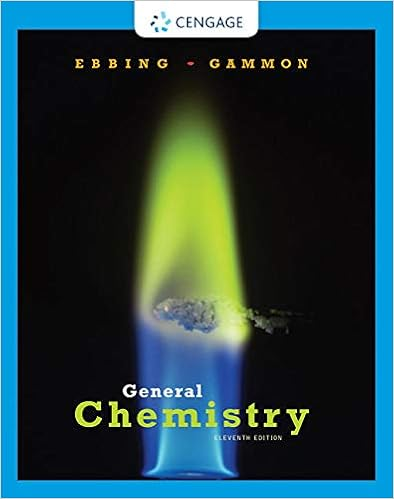Browse all Textbook Solutions

Course Hero member to access this document

End of preview. Want to read all 4 pages?

Course Hero member to access this document

Term
Fall
Professor
ZHAO
Tags
Atom, Moles, Chemical element, atomic number
##### We have textbook solutions for you!
The document you are viewing contains questions related to this textbook.The document you are viewing contains questions related to this textbook.
Chapter 3 / Exercise 3.27
Chemistry: The Molecular Science
Moore/StanitskiExpert Verified
•••